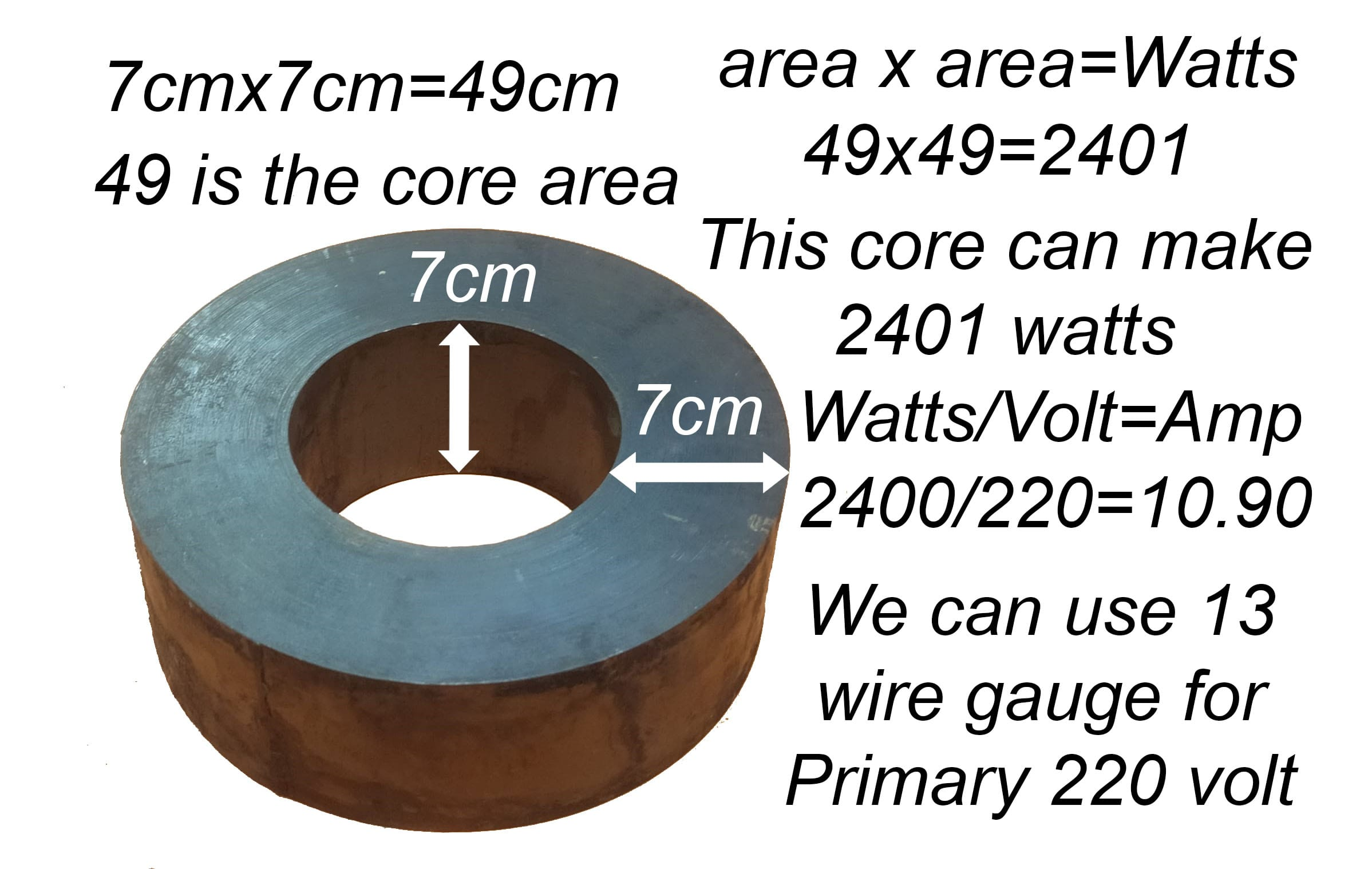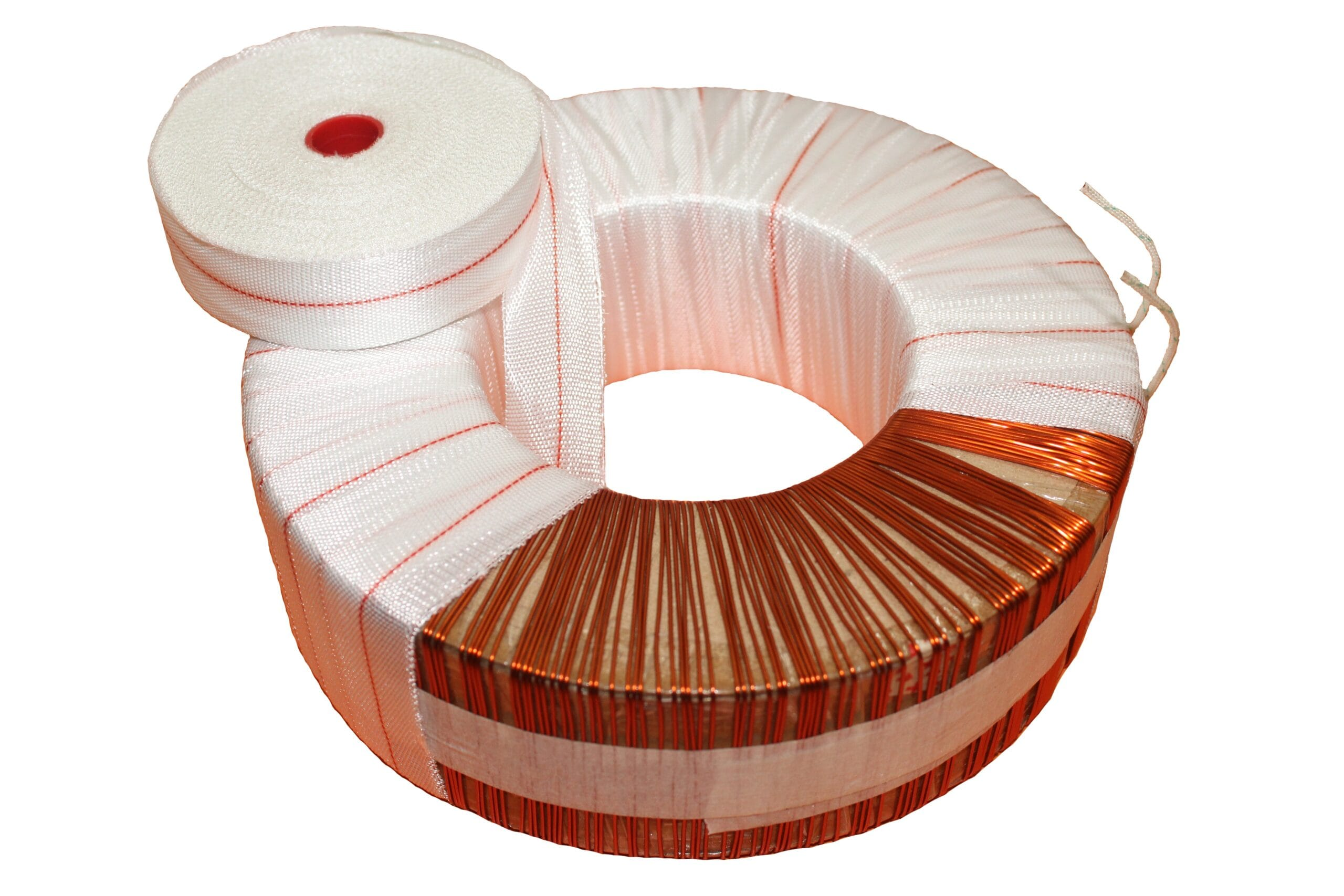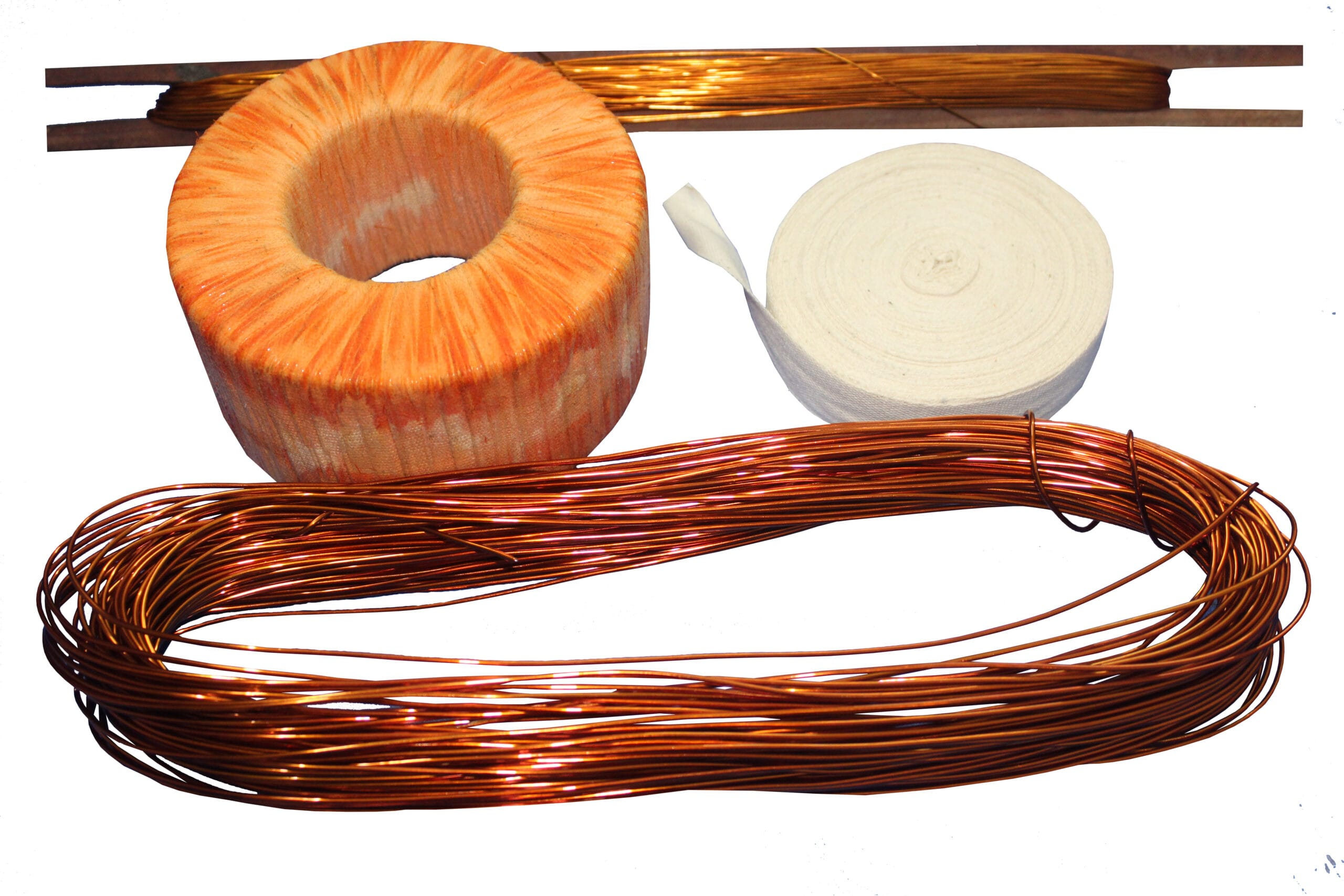# how to calculate transformer wire gauge

## how to calculate the transformer wire gauge?

how to calculate the transformer wire gauge? transformer winding has rules. we can get the watts according to the core area. like the core area is 20 then 20×20=400. 20 core area can make 400 watts. if the core area is 50 then. 50×50= 2500. 50 core area transformer can make 2500 watts.when we make a transformer. we have to use the transformer according to the amplifier’s watts. according to the transistor or IC. Also for battery chargers rules are the same. we have to follow the watts rules.Toroidal transformer and Classic transformer winding rules are same.Thanks a lot to be with us. If you want another post then please visit our website.

We have another post for you. like a Bass circuit diagram